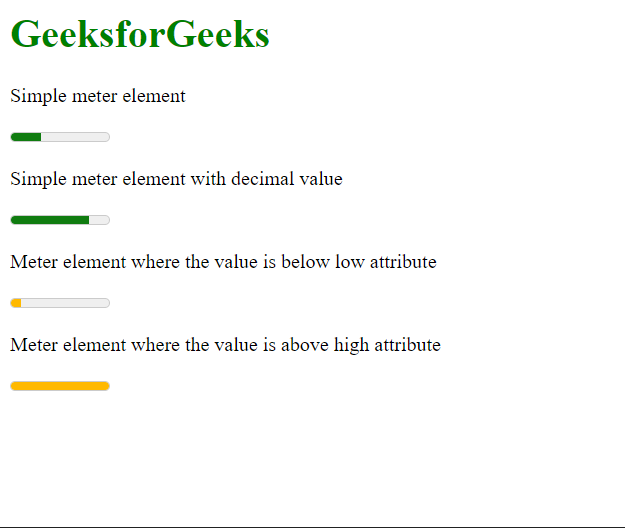# How to define scalar measurement within a given range in HTML5 ?

In this article, we will learn how to define an element that has a scalar measurement within a known range. It can be used to represent fractional data in a gauge making it easy for the reader to understand the values.

Approach:

We will use the <meter> element for defining the gauge that can measure the scalar value. This element has attributes that can be used to define the value to represent, the lower and higher limits of the range and the preferred range of values. The color of the meter changes depending on the value it represents and the browser style.

Syntax:

`<meter value="" min="" max=""></meter>`

The below example illustrates the <meter> element to define a scalar measurement.

Example:

## HTML

 ` ` `<``html``> ` ` `  `<``body``> ` `    ``<``h1` `style``=``"color: green;"``> ` `        ``GeeksforGeek ` `    `` ` ` `  `    ``<``p``>Simple meter element ` `    ``<``meter` `value``=``"3"` `min``=``"0"` `max``=``"10"`  `        ``low``=``"1"` `high``=``"8"``> ` `    `` ` ` `  ` `  `    ``<``p``>Simple meter element with decimal value ` `    ``<``meter` `value``=``"0.8"` `min``=``"0"` `max``=``"1"``> ` ` `  `    ``<``p``> ` `        ``Meter element where the value is ` `        ``below low attribute ` `    `` ` ` `  `    ``<``meter` `value``=``"1"` `min``=``"0"` `max``=``"10"`  `        ``low``=``"3"` `high``=``"9"``> ` `    `` ` ` `  `    ``<``p``> ` `        ``Meter element where the value is ` `        ``above high attribute ` `    `` ` ` `  `    ``<``meter` `value``=``"10"` `min``=``"0"` `max``=``"10"`  `        ``low``=``"3"` `high``=``"9"``> ` `    `` ` ` ` ` `  ` `

Output:Whether you're preparing for your first job interview or aiming to upskill in this ever-evolving tech landscape, GeeksforGeeks Courses are your key to success. We provide top-quality content at affordable prices, all geared towards accelerating your growth in a time-bound manner. Join the millions we've already empowered, and we're here to do the same for you. Don't miss out - check it out now!

Previous
Next